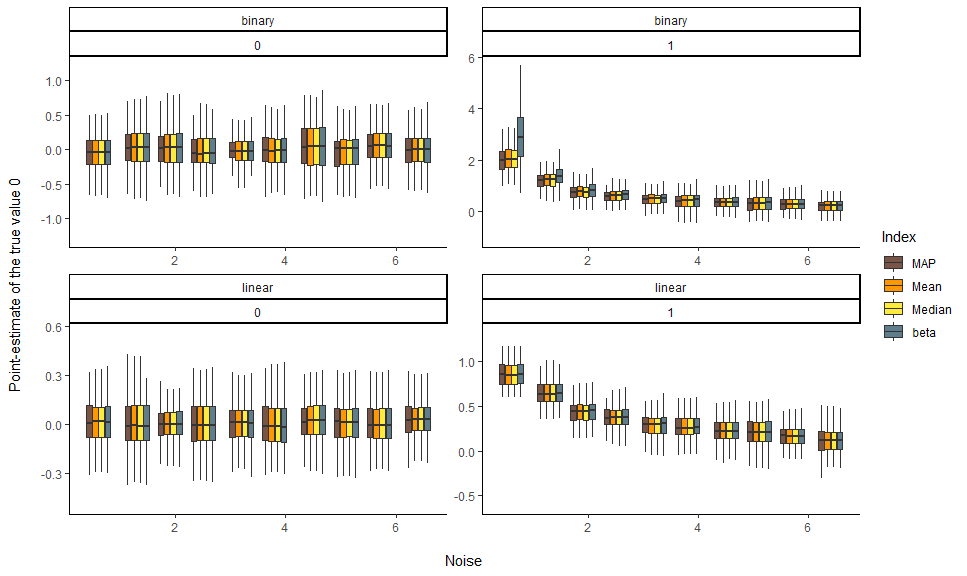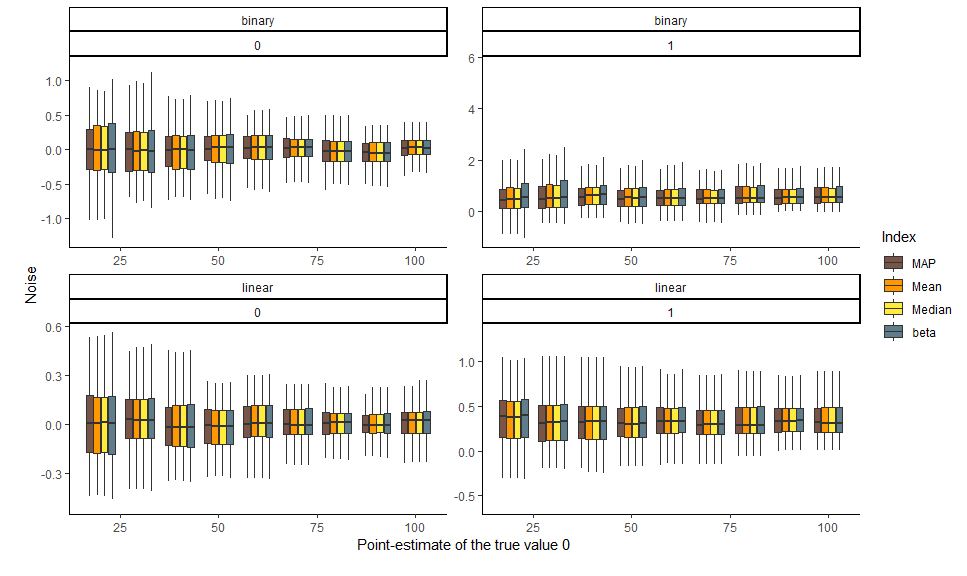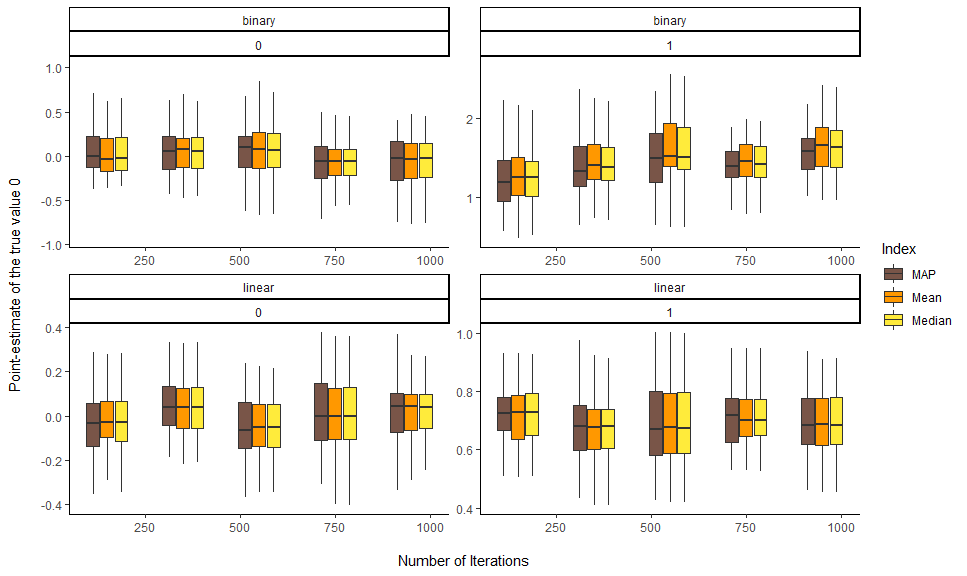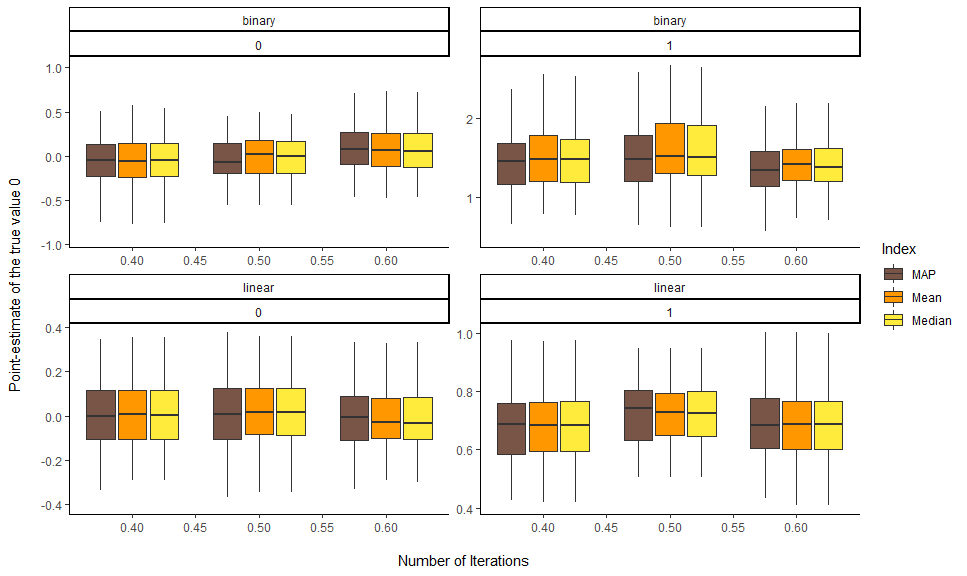# In-Depth 1: Comparison of Point-Estimates

This vignette can be referred to by citing the package:

# Effect Point-Estimates in the Bayesian Framework

## Introduction

One of the main difference between the Bayesian and the frequentist frameworks is that the former returns a probability distribution of each effect (i.e., parameter of interest of a model, such as a regression slope) instead of a single value. However, there is still a need and demand, for reporting or use in further analysis, for a single value (point-estimate) that best characterise the underlying posterior distribution.

There are three main indices used in the literature for effect estimation: the mean, the median or the MAP (Maximum A Posteriori) estimate (roughly corresponding to the mode (the “peak”) of the distribution). Unfortunately, there is no consensus about which one to use, as no systematic comparison has ever been done.

In the present work, we will compare these three point-estimates of effect between themselves, as well as with the widely known beta, extracted from a comparable frequentist model. With this comparison, we expect to draw bridges and relationships between the two frameworks, helping and easing the transition for the public.

## Experiment 1: Relationship with Error (Noise) and Sample Size

### Methods

The simulation aimed at modulating the following characteristics:

• Model type: linear or logistic.
• “True” effect (original regression coefficient from which data is drawn): Can be 1 or 0 (no effect).
• Sample size: From 20 to 100 by steps of 10.
• Error: Gaussian noise applied to the predictor with SD uniformly spread between 0.33 and 6.66 (with 1000 different values).

We generated a dataset for each combination of these characteristics, resulting in a total of `2 * 2 * 9 * 1000 = 36000` Bayesian and frequentist models. The code used for generation is avaible here (please note that it takes usually several days/weeks to complete).

``````library(ggplot2)
library(dplyr)
library(tidyr)

### Results

#### Sensitivity to Noise

``````df %>%
select(error, true_effect, outcome_type, beta, Median, Mean, MAP) %>%
gather(estimate, value, -error, -true_effect, -outcome_type) %>%
mutate(temp = as.factor(cut(error, 10, labels = FALSE))) %>%
group_by(temp) %>%
mutate(error_group = round(mean(error), 1)) %>%
ungroup() %>%
filter(value < 6) %>%
ggplot(aes(x = error_group, y = value, fill = estimate, group = interaction(estimate, error_group))) +
# geom_hline(yintercept = 0) +
# geom_point(alpha=0.05, size=2, stroke = 0, shape=16) +
# geom_smooth(method="loess") +
geom_boxplot(outlier.shape=NA) +
theme_classic() +
scale_fill_manual(values = c("beta" = "#607D8B", "MAP" = "#795548", "Mean" = "#FF9800", "Median" = "#FFEB3B"),
name = "Index") +
ylab("Point-estimate of the true value 0\n") +
xlab("\nNoise") +
facet_wrap(~ outcome_type * true_effect, scales="free") ``````#### Sensitivity to Sample Size

``````df %>%
select(sample_size, true_effect, outcome_type, beta, Median, Mean, MAP) %>%
gather(estimate, value, -sample_size, -true_effect, -outcome_type) %>%
mutate(temp = as.factor(cut(sample_size, 10, labels = FALSE))) %>%
group_by(temp) %>%
mutate(size_group = round(mean(sample_size))) %>%
ungroup() %>%
filter(value < 6) %>%
ggplot(aes(x = size_group, y = value, fill = estimate, group = interaction(estimate, size_group))) +
# geom_hline(yintercept = 0) +
# geom_point(alpha=0.05, size=2, stroke = 0, shape=16) +
# geom_smooth(method="loess") +
geom_boxplot(outlier.shape=NA) +
theme_classic() +
scale_fill_manual(values = c("beta" = "#607D8B", "MAP" = "#795548", "Mean" = "#FF9800", "Median" = "#FFEB3B"),
name = "Index") +
xlab("Point-estimate of the true value 0\n") +
ylab("\nNoise") +
facet_wrap(~ outcome_type * true_effect, scales="free")``````#### Statistical Modelling

We fitted a (frequentist) multiple linear regression to statistically test the the predict the presence or absence of effect with the estimates as well as their interaction with noise and sample size.

``````df %>%
select(sample_size, error, true_effect, outcome_type, beta, Median, Mean, MAP) %>%
gather(estimate, value, -sample_size, -error, -true_effect, -outcome_type) %>%
glm(true_effect ~ outcome_type / value * estimate * sample_size * error, data=., family="binomial") %>%
broom::tidy() %>%
select(term, estimate, p=p.value) %>%
filter(stringr::str_detect(term, 'outcome_type'),
stringr::str_detect(term, ':value')) %>%
mutate(
sample_size = stringr::str_detect(term, 'sample_size'),
error = stringr::str_detect(term, 'error'),
term = stringr::str_remove(term, "estimate"),
term = stringr::str_remove(term, "outcome_type"),
p = paste0(sprintf("%.2f", p), ifelse(p < .001, "***", ifelse(p < .01, "**", ifelse(p < .05, "*", ""))))) %>%
arrange(sample_size, error, term) %>%
select(-sample_size, -error) %>%
knitr::kable(digits=2) ``````
term estimate p
binary:value 2.72 0.05*
binary:value:Mean -0.18 0.93
binary:value:Median -0.08 0.97
binary:value:beta -1.08 0.56
linear:value 6.73 0.03*
linear:value:Mean -0.31 0.94
linear:value:Median -0.39 0.93
linear:value:beta -1.03 0.81
binary:value:Mean:error 0.03 0.94
binary:value:Median:error 0.01 0.98
binary:value:beta:error 0.19 0.64
binary:value:error -0.74 0.01*
linear:value:Mean:error 0.04 0.96
linear:value:Median:error 0.06 0.95
linear:value:beta:error 0.17 0.85
linear:value:error -1.91 0.00**
binary:value:Mean:sample_size 0.00 0.96
binary:value:Median:sample_size 0.00 0.97
binary:value:beta:sample_size 0.01 0.87
binary:value:sample_size 0.12 0.00***
linear:value:Mean:sample_size 0.01 0.93
linear:value:Median:sample_size 0.01 0.92
linear:value:beta:sample_size 0.02 0.88
linear:value:sample_size 0.26 0.00***
binary:value:Mean:sample_size:error 0.00 0.98
binary:value:Median:sample_size:error 0.00 0.97
binary:value:beta:sample_size:error 0.00 0.86
binary:value:sample_size:error 0.00 0.64
linear:value:Mean:sample_size:error 0.00 0.97
linear:value:Median:sample_size:error 0.00 0.96
linear:value:beta:sample_size:error 0.00 0.91
linear:value:sample_size:error 0.00 0.93

This suggests that, in order to delineate between the presence and the absence of an effect, compared to the frequentist’s beta:

• For linear Models;

• The mean, followed closely by the median, and the MAP estimate had a superior performance, altough not significantly.
• The mean, followed closely by the median, and the MAP estimate, were less affected by noise, altough not significantly.
• No difference for the sensitivity to sample size was found.
• For logistic models:

• The MAP estimate, followed by the median and the mean, estimate had a superior performance.
• The MAP estimate, followed by the median, and the mean, were less affected by noise, altough not significantly.
• The MAP estimate, followed by the mean, and the median, were less affected by sample size, altough not significantly.

## Experiment 2: Relationship with Sampling Characteristics

### Methods

The simulation aimed at modulating the following characteristics:

• Model type: linear or logistic.
• “True” effect (original regression coefficient from which data is drawn): Can be 1 or 0 (no effect).
• draws: from 10 to 5000 by step of 5 (1000 iterations).
• warmup: Ratio of warmup iterations. from 1/10 to 9/10 by step of 0.1 (9 iterations).

We generated 3 datasets for each combination of these characteristics, resulting in a total of `2 * 2 * 8 * 40 * 9 * 3 = 34560` Bayesian and frequentist models. The code used for generation is avaible here (please note that it takes usually several days/weeks to complete).

``df <- read.csv("https://raw.github.com/easystats/circus/master/data/bayesSim_study2.csv")``

### Results

#### Sensitivity to number of iterations

``````df %>%
select(iterations, true_effect, outcome_type, beta, Median, Mean, MAP) %>%
gather(estimate, value, -iterations, -true_effect, -outcome_type) %>%
mutate(temp = as.factor(cut(iterations, 5, labels = FALSE))) %>%
group_by(temp) %>%
mutate(iterations_group = round(mean(iterations), 1)) %>%
ungroup() %>%
filter(value < 6) %>%
ggplot(aes(x = iterations_group, y = value, fill = estimate, group = interaction(estimate, iterations_group))) +
geom_boxplot(outlier.shape=NA) +
theme_classic() +
scale_fill_manual(values = c("beta" = "#607D8B", "MAP" = "#795548", "Mean" = "#FF9800", "Median" = "#FFEB3B"),
name = "Index") +
ylab("Point-estimate of the true value 0\n") +
xlab("\nNumber of Iterations") +
facet_wrap(~ outcome_type * true_effect, scales="free") ``````#### Sensitivity to warmup ratio

``````df %>%
mutate(warmup = warmup / iterations) %>%
select(warmup, true_effect, outcome_type, beta, Median, Mean, MAP) %>%
gather(estimate, value, -warmup, -true_effect, -outcome_type) %>%
mutate(temp = as.factor(cut(warmup, 3, labels = FALSE))) %>%
group_by(temp) %>%
mutate(warmup_group = round(mean(warmup), 1)) %>%
ungroup() %>%
filter(value < 6) %>%
ggplot(aes(x = warmup_group, y = value, fill = estimate, group = interaction(estimate, warmup_group))) +
geom_boxplot(outlier.shape=NA) +
theme_classic() +
scale_fill_manual(values = c("beta" = "#607D8B", "MAP" = "#795548", "Mean" = "#FF9800", "Median" = "#FFEB3B"),
name = "Index") +
ylab("Point-estimate of the true value 0\n") +
xlab("\nNumber of Iterations") +
facet_wrap(~ outcome_type * true_effect, scales="free") ``````## Discussion

Conclusions can be found in the guidelines section.